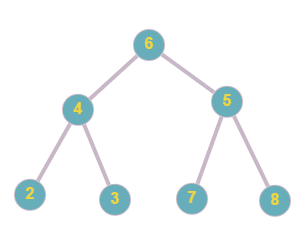# Count pairs of leaf nodes in a Binary Tree which are at most K distance apart

## Introduction

This article discusses how to count pairs of leaf nodes in a Binary Tree that are at most K distance apart. Such problems are the intermediate-level problems that a learner encounters while learning about binary trees.

## Problem Statement

You are given a binary tree Tree and an integer K. Count pair of leaf nodes which are at most K distance apart from each other.

## Example

Consider the given Binary Tree. And the value of K to be 4.Output: 6

Recommended: Before moving on to the solution approach please try to solve the problem on your own on Codestudio here - Number Of Good Leaf Nodes Pairs

## Approach and Explanation

As we can see from the above example, the valid lead node pairs at a distance of at most 4 are- (2,3), (2,7), (2,8), (3,7), (3,8), (7,8).

Here at most 4 means less than or equal to 4.

To do the required task, we have to first find all the child nodes, find the distance between them and return the value of pairs at a distance of at most K.

We solve the given problem in Java.

To implement the logic in Java, we do the following steps:

• Create an array (say res) that will store the number of leaf nodes at a distance i from the current node at res[i].

• We create two new arrays- leftST[] and rightST[].
• The element leftST[i] will store the number of leaf nodes in the left subtree, which are at a distance i from the current node.
• The element rightST[i] will store the number of leaf nodes in the right subtree, which are at a distance i from the current node.

• Once created, update the res[] array to store the distance between the nodes of the left subtree and the right subtree. That is, res[i+1] will store the distance between the left and the right subtree node at i using the expression:
res[i + 1] = leftST[i] + rightST[i]

• Now inside a nested for loop, create pairs of all the child nodes (l,r) and find their distance. If their distance comes out to be less than or equal to K, update the result variable as-
result += leftST[l] * rightST[r];
Basically, in this step, we are making the possible child node pairs (l,r) and checking the distance between them. If the distance comes out to be less than or equal to K, we increment the total count; that is the result.

• Return result.

## Java Implementation

``````class BTNode {
int data;
BTNode left, right;

public BTNode(int item)
{
data = item;
left = right = null;
}
}

public class ChildAtDistanceK {

BTNode root;
static int result;

static int[] findChildNodesK(BTNode root, int distK)
{
if (root == null)
return new int[distK + 1];

if (root.left == null && root.right == null) {
int[] res = new int[distK + 1];
res++;
return res;
}

int[] leftST = findChildNodesK(root.left, distK);

int[] rightST = findChildNodesK(root.right, distK);

int[] res = new int[distK + 1];

for (int i = res.length - 2; i >= 1; i--)
res[i + 1] = leftST[i] + rightST[i];

for (int l = 1; l < leftST.length; l++) {

for (int r = 0; r < rightST.length; r++) {

if (l + r <= distK) {
result += leftST[l] * rightST[r];
}
}
}

return res;
}

public static void main(String[] args)
{
ChildAtDistanceK tree = new ChildAtDistanceK();

/*
6
/  \
4     5
/  \      /  \
2   3   7   8
*/

tree.root = new BTNode(6);
tree.root.left = new BTNode(4);
tree.root.right = new BTNode(5);

tree.root.left.left = new BTNode(2);
tree.root.left.right = new BTNode(3);

tree.root.right.left = new BTNode(7);
tree.root.right.right = new BTNode(8);

result = 0;

int K = 4;

findChildNodesK(tree.root, K);

System.out.println(result + " pairs are at most at a distance of " + K + " from each other.");
}
}``````

OUTPUT

``6 pairs are at most at a distance of 4 from each other.``

## Complexities

### Time Complexity

In the given implementation, we traverse from one child node to another at a distance K. Thus time complexity is,

T(n) = O(N * K2),

where N is the number of nodes and K is the distance.

### Space Complexity

In the given implementation, we create an array that stores the number of nodes at distance K. Thus,

Space complexity = O(K+H),

where H is the height of the tree and K is the distance.

1. What does Post-Order Traversal mean?
Post-Order Traversal is a traversal technique where we first visit the left node, then the right node, then the root node. To learn more about Post Order Traversal and other traversal techniques, visit Binary Tree Traversals

2. What are leaf nodes?
A node having no child nodes is called a leaf node. Leaf nodes generally mark the end level of a node path in a tree. To learn more about leaf nodes and binary trees, visit Binary Tree.

## Key Takeaways

To summarize this article, we discussed how to count pairs of lead nodes in a Binary Tree which are at most K distance apart. We saw the problem statement, an example, an approach. We also saw the Java implementation of the approach and discussed its time and space complexity. We summed the article with some FAQs.

Improve your coding skills by practising various problems of various difficulty levels on our CodeStudio.

Learn about various topics like Web Technologies, Programing Fundamentals, Aptitude, DSA, and much more from our CN Library | Free Online Coding Resources.

Happy Coding!